# 机器学习：统计回归模型

(在苹果系统下，如果文章中的图片不能正常显示，请升级Safari浏览器到最新版本，或者使用Chrome、Firefox浏览器打开。)

1. 模型建立

y = θT * x

y = θ0 + θ1 * x1

我们都知道，房价跟房屋面积肯定是正相关的，但是并不是成正比的，具体的房价受制于多种因素，但最主要的因素是面积，这是毋庸置疑的，移动硬盘也是同样的道理。所以我们可以暂且忽略其他因素，抓住主要矛盾，研究房价与面积的关系，移动硬盘价格和容量的关系。

 面积 (m2) 40.1 63.5 65.13 80.15 85.62 91.22 95.56 98.36 100.56 120.32 总价 (万元) 39.86 65.99 64.98 80.1 85.65 92.11 95.1 99.12 101.23 119.85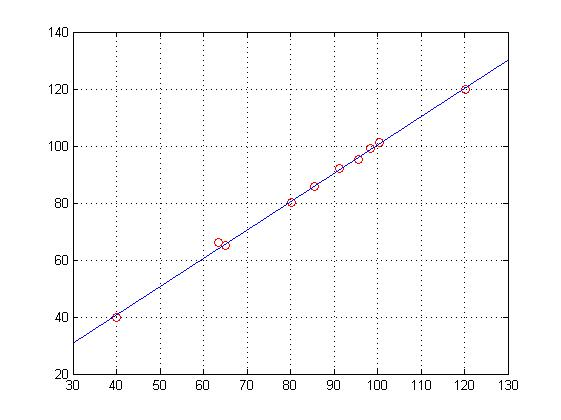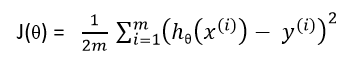1. 梯度下降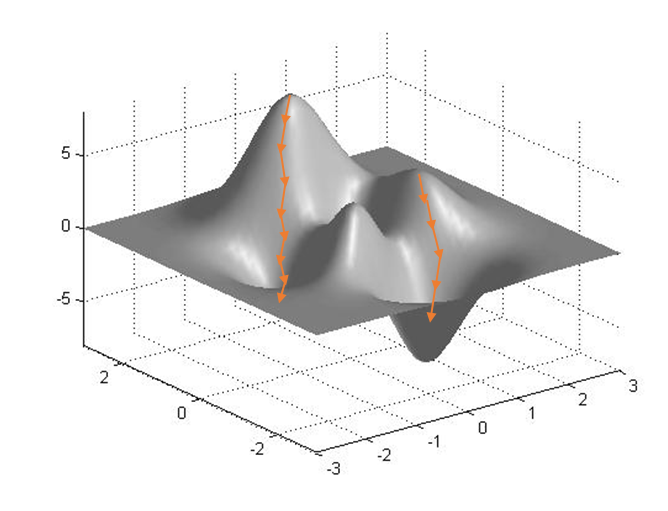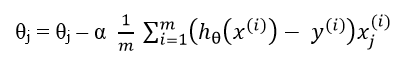(对于所有的j，需同时更新参数θj)

theta =

0.0118

1.0033

J =

0.3812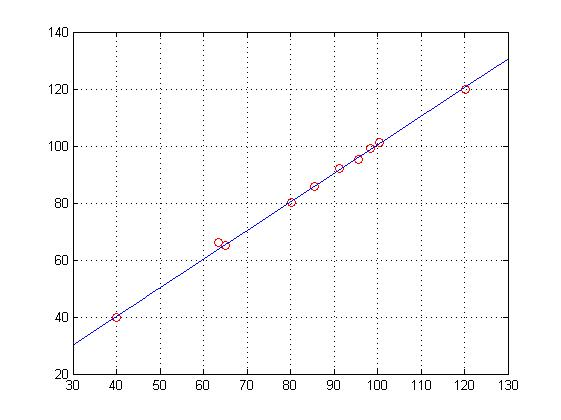Python代码 (Python3)

```import numpy as np
import matplotlib.pyplot as plt

# 数据
X=[40.10, 63.50, 65.13, 80.15, 85.62, 91.22, 95.56, 98.36, 100.56, 120.32]
Y=[39.86, 65.99, 64.98, 80.10, 85.65, 92.11, 95.10, 99.12, 101.23, 119.85]
X=np.matrix(X).T
Y=np.matrix(Y).T
# 初始化和准备工作
theta = np.zeros((2, 1), dtype = np.float) # 初始化拟合参数

# 一些梯度下降参数设置
iterations = 100 # 设置迭代次数
alpha = 0.0001 # 设置学习率
(m,n) = X.shape# 计算训练数据的数量

XX=np.hstack((np.ones((m, 1), dtype = np.float), X))# 在原来X的左边添加一行全为1的列向量

J = sum(np.power((XX * theta - Y),2)) / (2*m)#    计算初始的代价
# 开始梯度下降，结束后得到训练完毕的参数theta
J_history = []
for iter in range(0,iterations): # 这里不用while的原因是一旦出现发散的情况，程序将陷入死循环

theta = theta - alpha * 1/m * (XX.T * (XX * theta - Y))
# Save the cost J in every iteration
print(iter,'\n',theta,'\n')
cost = np.sum(np.power(XX * theta - Y,2)) / (2*m)
J_history.append(cost)#     XX(10,2)  theta(2,1)

# 显示训练结果和原始数据分布
J = np.sum(np.power((XX * theta - Y),2)) / (2*m)#     计算代价
print('Cost:',J)

x = np.arange(30, 1000) * 0.1
x=np.matrix(x).T
x_m = x.shape
xx=np.hstack((np.ones((x_m, 1), dtype = np.float), x))
y=xx*theta

plt.plot(x, y)
plt.show()
```

MATLAB (可运行于Octave)

1. polyfit()函数实现

```X=[40.10 63.50 65.13 80.15 85.62 91.22 95.56 98.36 100.56 120.32]';
Y=[39.86 65.99 64.98 80.10 85.65 92.11 95.10 99.12 101.23 119.85]';
plot(X,Y,'ro')
hold on
p=polyfit(X,Y,1)
x=[30:.1:130];
y=p(1)*x + p(2);
plot(x,y)
grid on
```

2.regress()函数实现

```X=[40.10 63.50 65.13 80.15 85.62 91.22 95.56 98.36 100.56 120.32]';
Y=[39.86 65.99 64.98 80.10 85.65 92.11 95.10 99.12 101.23 119.85]';
X=[ones(10,1), X];
[theta,bint,r,rint,stats]= regress(Y,X)
plot(X(11:20),Y,'ro')
hold on
x=[30:.1:130];
y=theta(1)+theta(2)*x;
plot(x,y)
grid on
```

3.梯度下降实现

```%% 数据
X=[40.10 63.50 65.13 80.15 85.62 91.22 95.56 98.36 100.56 120.32]';
Y=[39.86 65.99 64.98 80.10 85.65 92.11 95.10 99.12 101.23 119.85]';

%% 初始化和准备工作
theta = zeros(2, 1); % 初始化拟合参数
%theta = 2*ones(2, 1);
% 一些梯度下降参数设置
iterations = 100; % 设置迭代次数
alpha = 0.0001; % 设置学习率
[m,n] = size(X);  % 计算训练数据的数量
XX = [ones(m, 1), X]; % 在原来X的左边添加一行全为1的列向量
J = sum((XX * theta - Y).^2) / (2*m)     % 计算初始的代价

%% 开始梯度下降，结束后得到训练完毕的参数theta
J_history = zeros(iterations, 1);
for iter = 1:iterations % 这里不用while的原因是一旦出现发散的情况，程序将陷入死循环
theta = theta - alpha * 1/m * (XX' * (XX * theta - Y))
% Save the cost J in every iteration
J_history(iter) = sum((XX * theta - Y).^2) / (2*m);     % XX(10,2)  theta(2,1)
end

%% 显示训练结果和原始数据分布
J = sum((XX * theta - Y).^2) / (2*m)     % 计算代价

plot(X,Y,'ro')
hold on
x=[30:.1:130]';
[x_m,x_n]=size(x);
xx=[ones(x_m,1), x];    %添加一列全为1的向量
y=xx*theta;
plot(x,y)
grid on
```

 版权声明本博客的文章除特别说明外均为原创，本人版权所有。欢迎转载，转载请注明作者及来源链接，谢谢。本文地址: https://blog.ailemon.net/2017/02/10/machine-learningregression-statistic-model/ All articles are under Attribution-NonCommercial-ShareAlike 4.0

AI柠檬博主正在阿里云上出售域名 “y403.com”，感兴趣就快去看看吧

## “机器学习：统计回归模型”上的2条回复TuYuYu说：